Note: this document will print in an appropriately modified format (12 pages)

You know that an equilibrium constant expression looks something like K = [products] / [reactants]. But how do you translate this into a format that relates to the actual chemical system you are interested in? This lesson will show you how to write the equilibrium constant expressions that you will need to use when dealing with the equilibrium calculation problems in the chapter that follows this one.1  Pressures can express concentrations

Although we commonly write equilibrium quotients and equilibrium constants in terms of molar concentrations, any concentration-like term can be used, including mole fraction and molality. Sometimes the symbols
Kc , Kx , and Km are used to denote these forms of the equilibrium constant. Bear in mind that the numerical values of K’s and Q’s expressed in these different ways will not generally be the same.

Most of the equilibria we deal with in this course occur in liquid solutions and gaseous mixtures. We can express Kc values in terms of moles per liter for both, but when dealing with gases it is often more convenient to use partial pressures. These two measures of concentration are of course directly proportional: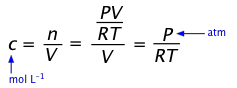(1-1)

so for a reaction A(g) → B(g) we can write the equilibrium constant as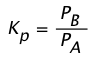(1-2)

Owing to interactions between molecules, especially when ions are involved, all of these forms of the equilibrium constant are only approximately correct, working best at low concentrations or pressures. The only equilibrium constant that is truly “constant” (except that it still varies with the temperature!) is expressed in terms of activities, which you can think of as “effective concentrations” that allow for interactions between molecules. In practice, this distinction only becomes important for equilibria involving gases at very high pressures (such as are often encountered in chemical engineering) and in ionic solutions more concentrated than about 0.001 M. We will not deal much with activities in this course.

For a reaction such as CO2(g) + OH(aq) → HCO3(aq) that involves both gaseous and dissolved components, a “hybrid” equilibrium constant is commonly used: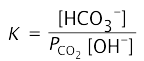(1-3)

Clearly, it is essential to be sure of the units when you see an equilibrium constant represented simply by "K".

### Converting between Kp and Kc

It is sometimes necessary to convert between equilibrium constants expressed in different units. The most common case involves pressure- and concentration equilibrium constants.

Note that when V is expressed in liters and P in atmospheres, R must have the value 0.08206 L-atm/mol K.)

The ideal gas law relates the partial pressure of a gas to the number of moles and its volume:

PV = nRT(1-4)

Concentrations are expressed in moles/unit volume n/V, so by rearranging the above equation we obtain the explicit relation of pressure to concentration:

P = (n/V)RT(1-5)

Conversely, c = (n/V) = P/RT(1-6)

... so a concentration [A] can be expressed as PA(RT).

For a reaction of the form  2 A → B + 3 C, we can write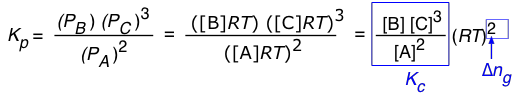(1-7)

So in general, assuming that all of the components are gases, the relationship between the two forms of K is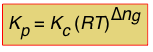(1-8)

in which Δng is the difference

moles of gas in products – moles of gas in reactants.

Note that for a reaction such as 2 HI(g) → H2(g) + I(g) in which Δng = 0, Kp and Kc will have identical values.

2  Don't show unchanging concentrations!
Substances whose concentrations undergo no significant change in a chemical reaction do not appear in equilibrium constant expressions.

How can the concentration of a reactant or product not change when a reaction involving that substance takes place? There are two general cases to consider.

#### The substance is also the solvent

This happens all the time in acid-base chemistry. Thus for the hydrolysis of the cyanide ion

CN+ H2O → HCN + OH

we write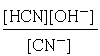in which no [H2O] term appears. The justification for this omission is that water is both the solvent and reactant, but only the tiny portion that acts as a reactant would ordinarly go in the equilibrium expression. The amount of water consumed in the reaction is so minute (because K is very small) that any change in the concentration of H2O from that of pure water (55.6 mol L–1) will be negligible.

Similarly, for the "dissociation" of water H2O → H+ + OH the equilibrium constant is expressed as the "ion product" Kw = [H+][OH].

But... be careful about throwing away H2O whenever you see it. In the esterification reaction

CH3COOH + C2H5OH → CH3COOC2H5 + H2O

that we discussed in a previous section, a [H2O] term must be present in the equilibrium expression if the reaction is assumed to be between the two liquids acetic acid and ethanol. If, on the other hand, the reaction takes place between a dilute aqueous solution of the acid and the alcohol, then the [H2O] term would not be included.

#### The substance is a solid or a pure liquid phase.

This is most frequently seen in solubility equilibria, but there are many other reactions in which solids are directly involved:

CaF2(s) → Ca2+(aq) + 2F(aq)

Fe3O4(s) + 4 H2(g) → 4 H2O(g) + 3Fe(s)

These are heterogeneous reactions (meaning reactions in which some components are in different phases), and the argument here is that concentration is only meaningful when applied to a substance within a single phase.

Thus the term [CaF2] would refer to the “concentration of calcium fluoride within the solid CaF2", which is a constant depending on the molar mass of CaF2 and the density of that solid. The concentrations of the two ions will be independent of the quantity of solid CaF2 in contact with the water; in other words, the system can be in equilibrium as long as any CaF2 at all is present.

Throwing out the constant-concentration terms can lead to some rather sparse-looking equilibrium expressions. For example, the equilibrium expression for each of the processes shown in the following table consists solely of a single term involving the partial pressure of a gas:

 1) CaCO3(s) → CaO(s) + CO2(g)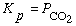Thermal decomposition of limestone, a first step in the manufacture of cement. 2) Na2SO4·10 H2O(s) Na2SO4(s) + 10 H2O(g)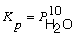Sodium sulfate decahydrate is a solid in which H2O molecules (“waters of hydration") are incorporated into the crystal structure.) 3) I2(s) → I2(g)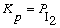sublimation of solid iodine; this is the source of the purple vapor you can see above solid iodine in a closed container. 4) H2O(l) → H2O(g)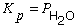Vaporization of water. When the partial pressure of water vapor in the air is equal to K, the relative humidity is 100%.

The last two processes 3 and 4 represent changes of state (phase changes) which can be treated exactly the same as chemical reactions.

In each of the heterogeneous processes shown in the table, the reactants and products can be in equilibrium (that is, permanently coexist) only when the partial pressure of the gaseous product has the value consistent with the indicated Kp. Bear in mind also that these Kp 's all increase with the temperature.

3  Evaluating equilibrium constants

Your ability to interpret the numerical value of a quantity in terms of what it means in a practical sense is an essential part of developing a working understanding of Chemistry. This is particularly the case for equilibrium constants, whose values span the entire range of the positive numbers.

Although there is no explicit rule, for most practical purposes you can say that equilibrium constants within the range of roughly 0.01 to 100 indicate that a chemically significant amount of all components of the reaction system will be present in an equilibrium mixture and that the reaction will be incomplete or “reversible”.

As an equilibrium constant approaches the limits of zero or infinity, the reaction can be increasingly characterized as a one-way process; we say it is “complete” or “irreversible”. The latter term must of course not be taken literally; the Le Châtelier principle still applies (especially insofar as temperature is concerned), but addition or removal of reactants or products will have less effect.

Although it is by no means a general rule, it frequently happens that reactions having very large equilibrium constants are kinetically hindered, often to the extent that the reaction essentially does not take place.

The examples in the following table are intended to show that numbers (values of K), no matter how dull they may look, do have practical consequences!

reaction K remarks
N2 + O2(g)→ 2 NO(g) 5×10–31 at 25°C,
0.0013 at 2100°C
These two very different values of K illustrate very nicely why reducing combustion-chamber temperatures in automobile engines is environmentally friendly.
3 H2(g)+ N2(g)→ 2 NH3(g) 7×105 at 25°C,
56 at 1300°C
See the discussion of this reaction in the section on the Haber process.
H2(g)→ 2 H(g) 10–36 at 25°C,
6×10–5 at 5000°
Dissociation of any stable molecule into its atoms is endothermic. This means that all molecules will decompose at sufficiently high temperatures.
H2O(g)→ H2(g)+ ½ O2(g) 8×10–41 at 25°C You won’t find water a very good source of oxygen gas at ordinary temperatures!
CH3COOH(l)
2 H2O(l)+ 2 C(s)
Kc = 1013 at 25°C This tells us that acetic acid has a great tendency to decompose to carbon, but nobody has ever found graphite (or diamonds!) forming in a bottle of vinegar. A good example of a super kinetically-hindered reaction!
4  Do equilibrium constants have units?

The short answer is yes, but for most practical purposes, you can ignore them.

The equilibrium expression for the synthesis of ammonia

3 H2(g)+ N2(g) → 2 NH3(g)

can be expressed asorso Kp and Qp for this process would appear to have units of atm–1, and Kc and Qc would be expressed in mol–2 L2. And yet these quantities are often represented as being dimensionless. Which is correct? The answer is that both forms are acceptable. There are some situations (which you will encounter later) in which K’s must be considered dimensionless, but in simply quoting the value of an equilibrium constant it is permissible to include the units, and this may even be useful in order to remove any doubt about the units of the individual terms in equilibrium expressions containing both pressure and concentration terms. In carrying out your own calculations, however, there is rarely any real need to show the units.

The reason that common practice hides the units in equilibriuim expressions is because the concentration or pressure terms that go into them are really ratios having the forms (n mol L–1)/(1 mol L–1) or (n atm)/(1 atm) in which the unit quantity in the denominator refers to the standard state of the substance; thus the units always cancel out. (But first-year students are not expected to know this!)

For substances that are liquids or solids, the standard state is just the concentration of the substance within the liquid or solid, so for something like CaF(s), the term going into the equilibrium expression is [CaF2]/[CaF2] which cancels to unity; this is the reason we don’t need to include terms for solid or liquid phases in equilibrium expressions. The subject of standard states would take us beyond where we need to be at this point in the course, so we will simply say that the concept is made necessary by the fact that energy, which ultimately governs chemical change, is always relative to some arbitrarily defined zero value which, for chemical substances, is the standard state.

5  How the form of the reaction equation affects K

It is important to remember that an equilibrium quotient or constant is always tied to a specific chemical equation, and if we write the equation in reverse or multiply its coefficients by a common factor, the value of Q or K will change.

The rules are very simple:

•  Writing the equation in reverse will invert the equilibrium expression;

•  Multiplying the coefficients by a common factor will raise Q or K to the corresponding power.

Here are some of the possibilities for the reaction involving the equilibrium between gaseous water and its elements:

 2 H2 + O2→ 2 H2O 10 H2 + 5 O2 → 10 H2O H2 + ½ O2 → H2O H2O → H2 + ½ O2### Equilibrium constant for a sequence of reactions

Many chemical changes can be regarded as the sum or difference of two or more other reactions. If we know the equilibrium constants of the individual processes, we can easily calculate that for the overall reaction according to this rule:

The equilibrium constant for the sum of two or more reactions is the product of the equilibrium constants for each of the steps.

6  Heterogeneous reactions

Reactions involving more than one phase are said to be heterogeneous. Some examples:

 Fe(s) + O2(g) → FeO2(s) air-oxidation of metallic iron (formation of rust) CaF2(s) → Ca2+(aq) + F+(aq) dissolution of calcium fluoride in water H2O(s) → H2O(g) sublimation of ice (a phase change) NaHCO3(s) + H+(aq) → CO2(g) + Na+(aq) + H2O(g) formation of carbon dioxide gas from sodium bicarbonate when water is added to baking powder (the hydrogen ions come from tartaric acid, the other component of baking powder.)

Bear in mind that terms related to condensed phases (solids and liquids) do not appear in equilibrium expressions.

### Vapor pressures of solid hydrates

A particularly interesting type of heterogeneous reaction is one in which a solid is in equilibrium with a gas. The sublimation of ice illustrated in the above table is a very common example. The equilibrium constant for this process is simply the partial pressure of water vapor in equilibrium with the solid— the vapor pressure of the ice.

Many common inorganic salts form solids which incorporate water molecules into their crystal structures. These water molecules are usually held rather loosely and can escape as water vapor.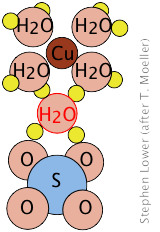Copper(II) sulfate, for example forms a pentahydrate in which four of the water molecules are coordinated to the Cu2+ ion, while the fifth is hydrogen-bonded to
to the sulfate (SO42–) ions of two adjacent SO42– units, joining them together in a chain.

If the intensely blue pentahydrate CuSO4·5H2O is heated, the waters of hydration can be driven off, changing it into the colorless anhydrous salt:

CuSO4·5H2O(s) → CuSO4 (s) + 5 H2O(g)

Careful examination of this process reveals that it takes place in a succession of smaller steps which can be carried out at the temperatures indicated: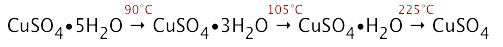But even at lower temperatures, each hydrate can slowly lose moisture in dry air. Thus if the solid pentahydrate is placed in a closed container at room temperature, it will slowly reach equilibrium with the trihydrate and water vapor:

CuSO4·5H2O(s) → CuSO4·3H2O(s) + 3 H2O(g)

Eventually, of course, the trihydrate will equilibrate with the monohydrate, and the latter with the anhydrous salt.  But these reactions are so slow at room temperature, that each equilibrium can be studied individually.

It is important to understand that it is meaningless to assign a vapor pressure or an equilirium constant to an individual hydrate in which dehydration occurs in multiple steps, as is the case for CuSO4·5H2O; for equilibrium to be attained in these stepwise dehydrations, two solids, plus water vapor, must be simultaneously present.

However, it is not uncommon to see, both in the literature and in textbooks, tables such as the following, in which the "vapor pressure of the hydrate" is just the one associated with the first step. Although this can be misleading to the student, there is some practical utility this practice, since the fully hydrated salts are usually usually the only ones commonly encountered and readily available.

name formula vapor pressure, torr
25°C 30°C
sodium sulfate decahydrate Na2SO4·10H2O 19.2 25.3
copper(II) sulfate pentahydrate CuSO4·5H2O 7.8 12.5
calcium chloride monohydrate CaCl2·H2O 3.1 5.1
(water) H2O 23.5 31.6

The ability of a hydrated salt in equilibrium with its less-hydrated form to maintain a constant partial pressure of water vapor in an enclosed space is sometimes exploited as a simple means of humidity control in laboratory experiments relating to materials science or biology. (For more on this, see here.)

#### Efflorescence and deliquescence

If a hydrate is exposed to air in which the partial pressure of water vapor is less than its vapor pressure, the reaction will proceed to the right and the hydrate will lose moisture.

Loss of water usually causes a breakdown in the structure of the crystal; this is commonly seen with sodium sulfate decahydrate, whose vapor pressure is sufficiently large that it can exceed the partial pressure of water vapor in the air when the relative humidity is low. What one sees is that the well-formed crystals of the decahydrate undergo deterioration into a powdery form, a phenomenon known as efflorescence.

When a solid is able to take up moisture from the air, it is described as hygroscopic. A small number of anhydrous solids that have low vapor pressures not only take up atmospheric moisture on even the driest of days, but will become wet as water molecules are adsorbed onto their surfaces; this is most commonly observed with sodium hydroxide and calcium chloride. With these solids, the concentrated solution that results continues to draw in water from the air so that the entire crystal eventually dissolves into a puddle of its own making; solids exhibiting this behavior are said to be deliquescent.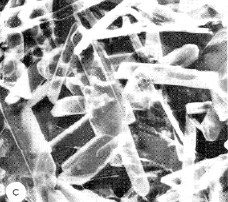One of the first hydrates investigated in detail was calcium sulfate hemihydrate (CaSO4·½ H2O) which LeChâtelier (he of the “principle”) showed to be the hardened form of CaSO4 known as plaster of Paris. Anhydrous CaSO4 forms compact, powdery crystals, whereas the elongated crystals of the hemihydrate bind themselves into a cement-like mass that makes this material useful for making art objects, casts for immobilizing damaged limbs, and as a construction material (fireproofing, drywall.)

What you should be able to do

Make sure you thoroughly understand the following essential ideas which have been presented above. It is especially imortant that you know the precise meanings of all the highlighted terms in the context of this topic.

• The equilibrium quotient Q is the value of the equilibrium expression of a reaction for any arbitrary set of concentrations or partial pressures of the reaction components.
• The equilibrium constant K is the value of Q  when the reaction is at equilibrium. K has a unique value for a given reaction at a fixed temperature and pressure.
• Q and K can be expressed in terms of concentrations, partial pressures, or, when appropriate, in some combination of these.
• For a reaction in which all the components are gases, Qc and Kc will have different values except in the special case in which the total number of moles of gas does not change.
• Concentration terms for substances whose concentrations do not change in the reaction do not appear in equilibrium expressions. The most common examples are [H2O] when the reaction takes place in aqueous solution (so that [H2O] is effectively constant at 55.6 M), and in heterogeneous reactions involving solids, in which the concentration of the solid is determined by the density of the solid itself.
• A reaction whose equilibrium constant is in the range of about 0.01 to 100 is said to be incomplete or [thermodynamically] reversible.
• Q and K are conventionally treated as dimensionless quantities, and need not ordinarily have units associated with them.
• Heterogeneous reactions are those in which two or more phases are involved; homogeneous reactions take place in a single phase. A common type of heterogeneous reaction is the loss of water of crystallization by a solid hydrate such as CuSO4·5H2O.
• The equilibrium expression can be manipulated and combined in the following ways:
• If the reaction is written in reverse, Q becomes Q–1;
• If the coefficients of an equation are multiplied by n, Q becomes Qn;
• Q for the sum of two reactions (that is, for two reactions that take place in sequence) is the product (Q1)(Q2).
Concept Map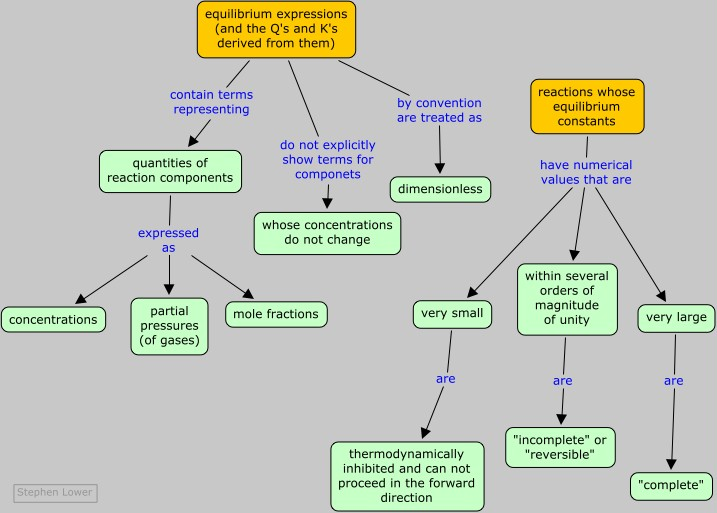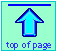Chem1 Equilibrium Expressions explains how to write formulas for equilibrium constants/quotients for various types of reactions. It is Part 4 of a 5-part set of lessons on Chemical Equilibrium for a course in General Chemistry. It is part of the General Chemistry Virtual Textbook, a free, online reference textbook for General Chemistry by of

Simon Fraser University .

This chapter covers the following topics: Converting between gas pressures and concentrations, treating unchanging concentrations, do equilibrium constants have units?, how the form of the reaction equation affects K or Q, Heterogeneous reactions and solid hydrates. It can be accessed directly at .